# Money split

Anton and Ferko got 2,500 euros together. The amount was split in a 1:4 ratio. How much did each get?

a =  500 Eur
f =  2000 Eur

### Step-by-step explanation: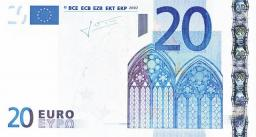Did you find an error or inaccuracy? Feel free to write us. Thank you!Tips to related online calculators
Check out our ratio calculator.

## Related math problems and questions:

• Euros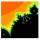Michal, Peter, John and Lenka got together 2,400 euros. They share an amount in ratio 2:6:4:3. How many got each of them?
• Moneys in triple ratioMilan, John and Lili have a total 344 euros. Their amounts are in the ratio 1:2:5. Determine how much each of them has?
• AndreAndre, Thomas, and Ivan split 88 postage stamps in a 2:5:4 ratio. How much did Thomas get?
• Unknown amount of money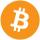Damian and Denis split an unknown amount in the ratio of 5:4. Damian got six euros more than Denis. Calculate an unknown amount. Determine how much money got Damian and how Denis.
• Division of moneyCalculate how many euros have Matthew, Miriam, Lucy, Michael, Janka when together have 2,700 euros and the amounts are at a ratio of 1:5:6:7:8.
• Divide moneyDivide 1200 USD at a ratio of 1:2:3:4:5:6:9:10
• ErikThree friends Peter, Erik and Milan shared 420 euros in a ratio of 1: 2: 3. How many euros did Erik get?
• Composite ratioJakub, Aneta, and Lenka divided 1342 USD in the ratio 5/2: 3/10: 1/4. How much did Lenka take?
• Revenue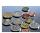Mr. Robert and Mr. Norbert get together € 1400. The revenue split in the ratio of 4:3, depending upon each work. How many got each of them?
• Dividing moneyMilan divided 360 euros in the ratio 4: 5, Erik in the ratio 500: 625. How many euros did the individual parts of Milan and how many Erik?
• Ratio6 numbers are in the ratio 1:5:1:5:5:5. Their sum is 242. What are the numbers?
• Division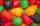Three siblings Helena, Oliver and George split the bag with candies on merit in the ratio 6:1:4. How many candies should each get if in bag were 88?
• GardenThe rectangular garden has dimensions of 27 m and 30 m. Peter and Katka split it in a ratio of 4:5. How many square meters did Katkin measure part of the garden?
• Kilo candyOne kilogram of chocolate candy cost 10 euros. Kate buys 250 grams and had the 8 pack of candy. How much does cost one bonbon?
• PeppersIn the box are yellow (a), green (b), and red (c) peppers. Their amount is in a ratio of 2:4:1. Most are yellow peppers and green the least. Calculate the number of peppers each type if the total number of peppers is 70.
• Dividing moneyImrich, Daniel and Dezider shared an unknown amount in the ratio 1:2:4, where Dezider received 750 euros more than Imrich and Daniel got half as much as Dezider. Determine an unknown amount of money and determine the amounth that got Imrich, Daniel and De
• Split between 4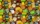There are 12 1/4 pounds of candy canes to split between four classes evenly. How many pounds of the candy canes will each class get?